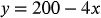1
Chapter
1.2
Section
35
Page
12a
Problem

# Problem 12a

## Chapter 1 | Section 1.2 | Page 35Textbook ExpertVerified Tutor
14 Oct

#### Given information

Given the equation -. for sketching the graph.

#### Step-by-step explanation

Step 1.

For sketching the graph, the coordinates of x and y are calculated.

The equationis used here to calculate the coordinates.

 x 0 50 20 y 200 0 120

Further, the points are plotted on the graph.